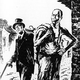# Difference % between PRICE and VWAP V2

2868 visualizzazioni
2868
Shows difference between price and daily/weekly/monthly/hourly/whatever VWAP .
In v2:
option to color bars
average percentual difference of custom period
histogram changes color depending on which levels it's at
Levels/period/color etc can be customized.

Use these inputs in the timeframe box:
M - month
W - week
D - day
2D (or 3W or 2M or whatever) = 2 Days (or three week or 2 months and so on)
60= 1 H
240= 4 H
5 = 5 min
and so on.

btw, vaguely remembering reading somewhere that the big players like to make their entry at 4% difference.
```//@version=2
study(title="Percent difference between price and vwap")
BarcOn=input(false, title="Color bars/Candles?")
len=input(36)
TimeFrame = input('D')
start = security(tickerid, TimeFrame, time)

newSession = iff(change(start), 1, 0)

vwapsum = iff(newSession, hl2*volume, vwapsum+hl2*volume)
volumesum = iff(newSession, volume, volumesum+volume)
v2sum = iff(newSession, volume*hl2*hl2, v2sum+volume*hl2*hl2)
myvwap = vwapsum/volumesum

src=input(close)
xSMA = myvwap
nRes = abs(src - xSMA) * 100 / src

nRes3 = sma(nRes,len)
plot(nRes3, color=blue, style=areabr,transp=90, histbase=0, title="Average")
level1=input(1.28)
level2=input(2.1)
level3=input(2.5)
level4=input(3.09)
level5=input(4.1)
color2=nRes>level1 and nRes<level2?navy: nRes>level2 and nRes<level3?blue: nRes>level3 and nRes<level4?orange: nRes>level4 and nRes<level5?red: nRes>level5?maroon: na
color=nRes>level1 and nRes<level2?navy: nRes>level2 and nRes<level3?blue: nRes>level3 and nRes<level4?orange: nRes>level4 and nRes<level5?red: nRes>level5?maroon: gray

plot(nRes, style=histogram,color=color)
barcolor(BarcOn?color2:na)
hline(0, title="Base Line", color=aqua, linestyle=solid)
a=hline(level1, title="1", color=aqua, linestyle=dotted)
b=hline(level2, title="2", color=blue, linestyle=dotted)
c=hline(level3, title="3", color=orange, linestyle=dotted)
d=hline(level4, title="4", color=red, linestyle=dotted)
e=hline(level5, title="5", color=maroon, linestyle=dotted)```